# How to interact with barbatruc ?¶

## LAUNCHING¶

To have the results :

Go to the folder where your file is :

```cd folder/
```

To launch the simulation :

```python3 cfd_cylinder.py
```

and you get :

```	.  Rectangular Grid.
=======================

y_max: 0.5m, nodes: 100
+----------------------------+
|                            |
|                            |
|                            |
|                            |
|                            |
+----------------------------+
(0,0)                        x_max: 1.0m, nodes: 200

periodic
+----------------------------+
|                            |
inlet
|                          outlet
|                            |
|                            |
+----------------------------+
periodic

.  Fields stats
=======================
.  min|avg|max
vel_u : 1.0 | 1.0 | 1.0
vel_v : 0.1 | 0.10000000000000002 | 0.1
scal : 0.0 | 0.0 | 0.0
press : 0.0 | 0.0 | 0.0

===============================

Iteration 1/200, Time :, 0.02s
Reynolds : 111.92422560738474

.  Fields stats
=======================
.  min|avg|max
vel_u : 0.12019082043493529 | 0.9940530128736734 | 1.3605910402433394
vel_v : -0.3009720354811324 | 0.09675753736792093 | 0.4664584367055691
scal : 0.0 | 0.0 | 0.0
press : -2.2893733111942414 | 0.0 | 1.7798464788156056

===============================

Iteration 2/200, Time :, 0.04s
Reynolds : 112.09757332022016

.  Fields stats
=======================
.  min|avg|max
vel_u : 0.023893464480471707 | 0.9953864667605914 | 1.3804213543256483
vel_v : -0.329530409060508 | 0.0944418335727916 | 0.5040636022356708
scal : 0.0 | 0.0 | 0.0
press : -1.4163675489683598 | -7.105427357601002e-18 | 1.4278708080524152

===============================

Iteration 3/200, Time :, 0.06s
Reynolds : 111.90076036667641

.  Fields stats
=======================
.  min|avg|max
vel_u : -0.06308165801559718 | 0.9935347780711958 | 1.3528315628913046
vel_v : -0.3441370891819653 | 0.0922008027913569 | 0.5008225700261179
scal : 0.0 | 0.0 | 0.0
press : -1.276280378390031 | 2.1316282072803004e-18 | 1.2726099477265453

===============================
...
```

To interrupt the simulation :

```ctrl + c
```

To know how much time the simulation takes :

```time python3 cfd_cylinder.py

real	1m10.974s
user	1m49.836s
sys	0m14.051s
```

### Remarks :¶

In the python file, a function is defined to compute the results :

```def cfd_cylinder(nsave):
```

time_step is the observation time and not the one which respect the CFL.

nsave is the number of iteration :

```time_step = t_end/nsave

t_end = 4.0 * lenght / vel
```

## CHANGING CASE¶

### Trick¶

Comment what you don’t want to use.

To change the solver :

``` #solver = Lattice(dom, max_vel=2*vel)
solver = NS_fd_2D_explicit(dom, obs_ib_factor=0.9)
```

obs_ ib _factor can be considered as a porosity factor of the obstacle (cylinder).

Take an example with known results, apply the two solvers, choose the one which is better for this case.

To change the boundary conditions :

```dom.switch_bc_xmin_inlet(vel_u=vel)
#dom.switch_bc_xmax_outlet()
dom.switch_bc_ymax_wall_noslip()
dom.switch_bc_ymin_wall_noslip()
```

### To change the case :¶

The default parameters are in fluid_domain.py. If they are specified in one of the examples (cfd_poiseuille.py, cfd_ lid _driven.py, cfd_cylinder.py) then those values are used.

1. Change the dimensions with delta_x = dimy/number of cells :

``` dom = DomainRectFluid(dimx=0.82, dimy=0.61, delta_x=0.02)
```
2. To have the Reynolds number that you want either change the velocity or the viscosity :

For Re = 20 and default viscosity nu_ = 1.0 :

```vel = 32.7869
```

Be aware that by changing the velocity, you need to change the time : t_end = dimx/vel.

or with default velocity vel = 0.0001

```dom = DomainRectFluid(dimx=0.82, dimy=0.61, delta_x=0.02, nu = 0.000003)
```

## VIEWING¶

Results for a flow around a cylinder

### Fields at Small Reynolds Number :¶

```dom.show_fields()
```
• vel_u : horizontal velocity
• vel_v : vertical velocity
• press : pressure
• scal : passive scalar which can simulate the trajectory of a leak of pollutant or the dissipation of a perfume in a room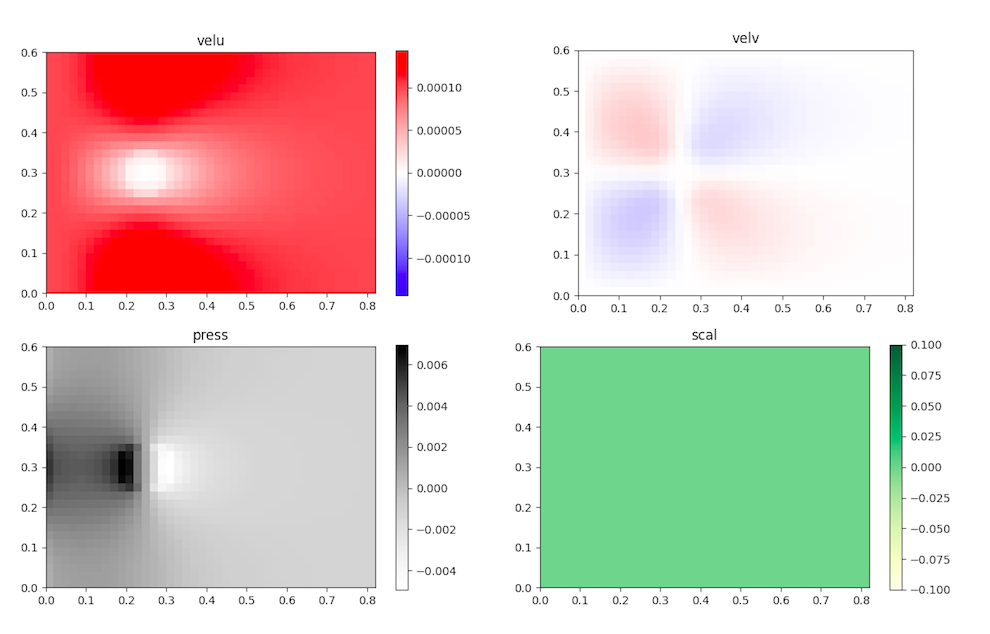cylinderfields

### The flow output at Small Reynolds Number :¶

```dom.show_flow()
```
• velocity field with its intensity (color code)
• streamlines (blue vectors)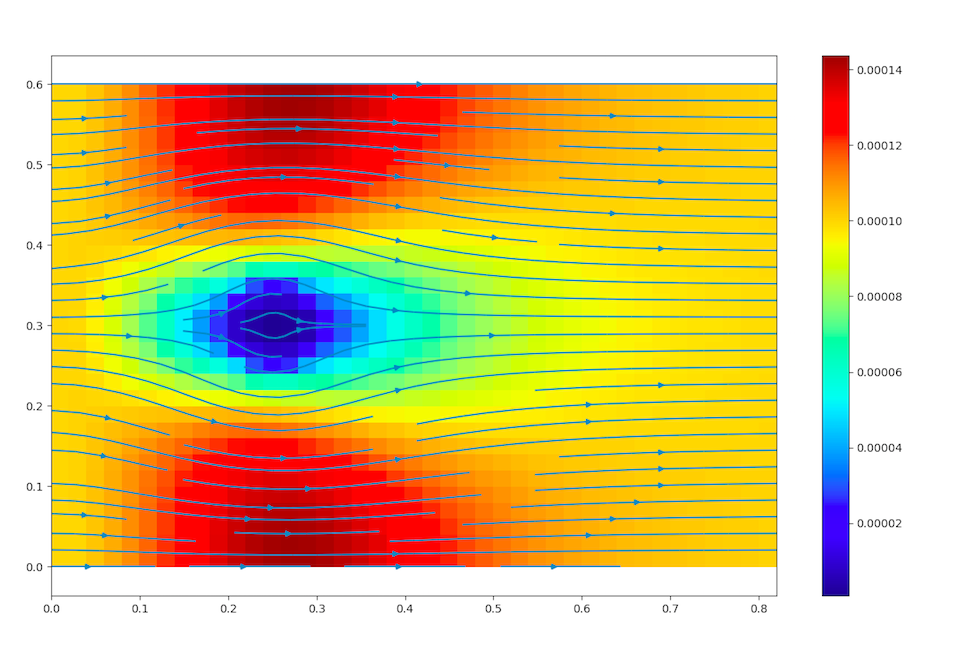cylinderflow

### The velocity profile at Small Reynolds Number :¶

To get the profile at x=cste : change the value of xtgt.

```dom.show_profile_y(xtgt=0.25, dimx=0.82, delta_x=0.02)
```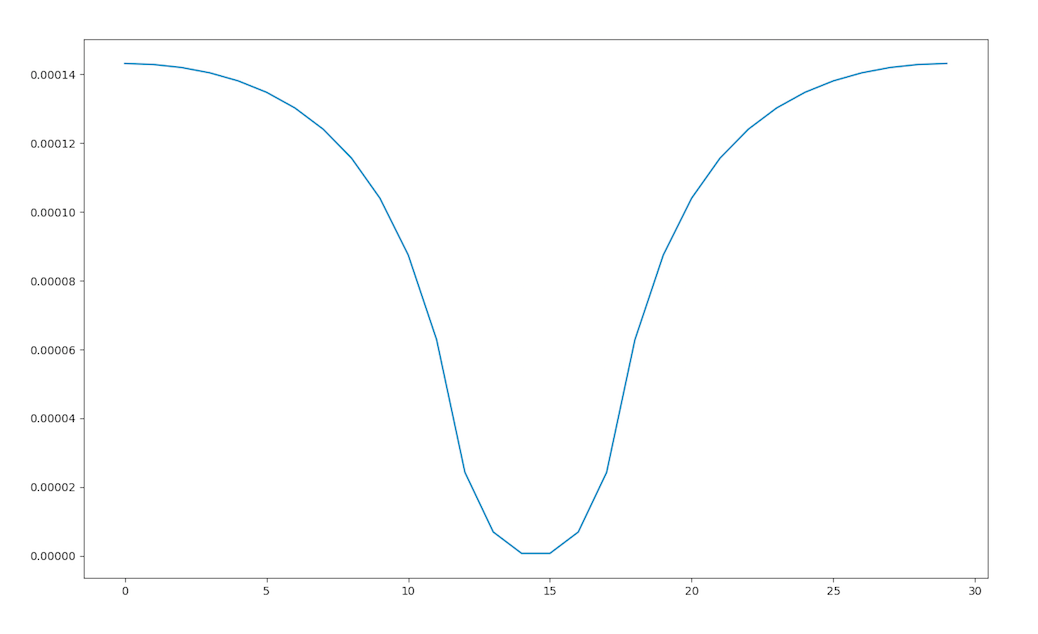cylinderprofile

### The monitors at High Reynolds Number :¶

This kind of monitoring only works with he Navier-Stokes solver; the LBM solver is not ready yet.

```barbatruc monitor solver
```

The solver monitoring gives the following data in terms of time:

• the velocity for the number of step of Poisson
• the Poisson final residual
• the Maximum velocity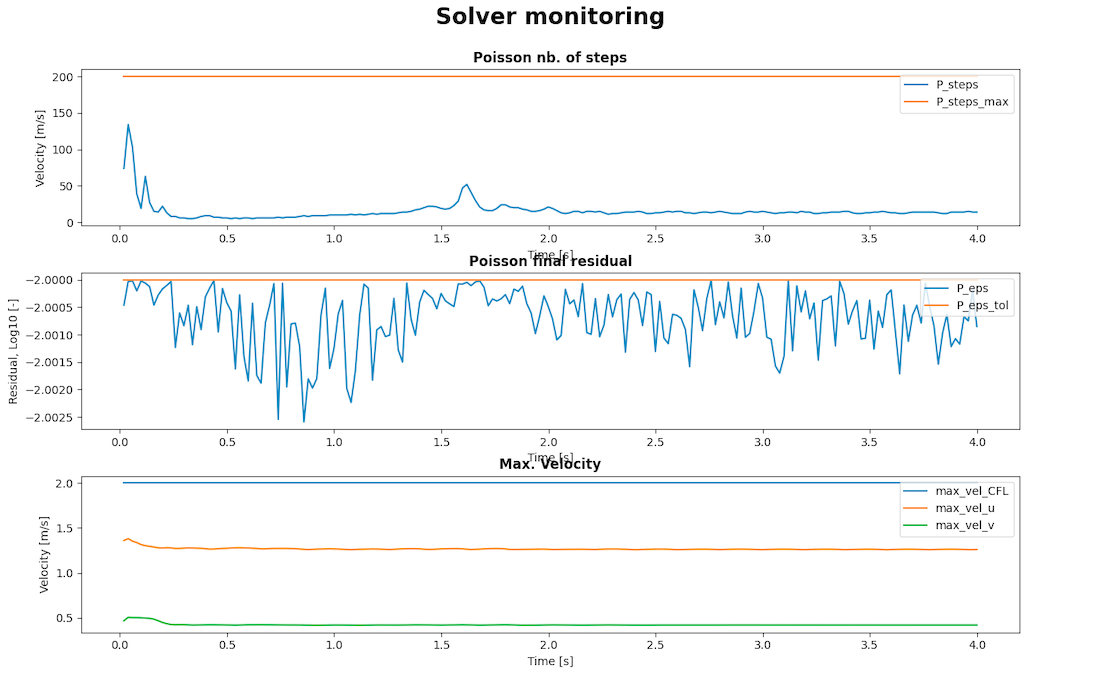karmanmonitor

```barbatruc monitor glob
```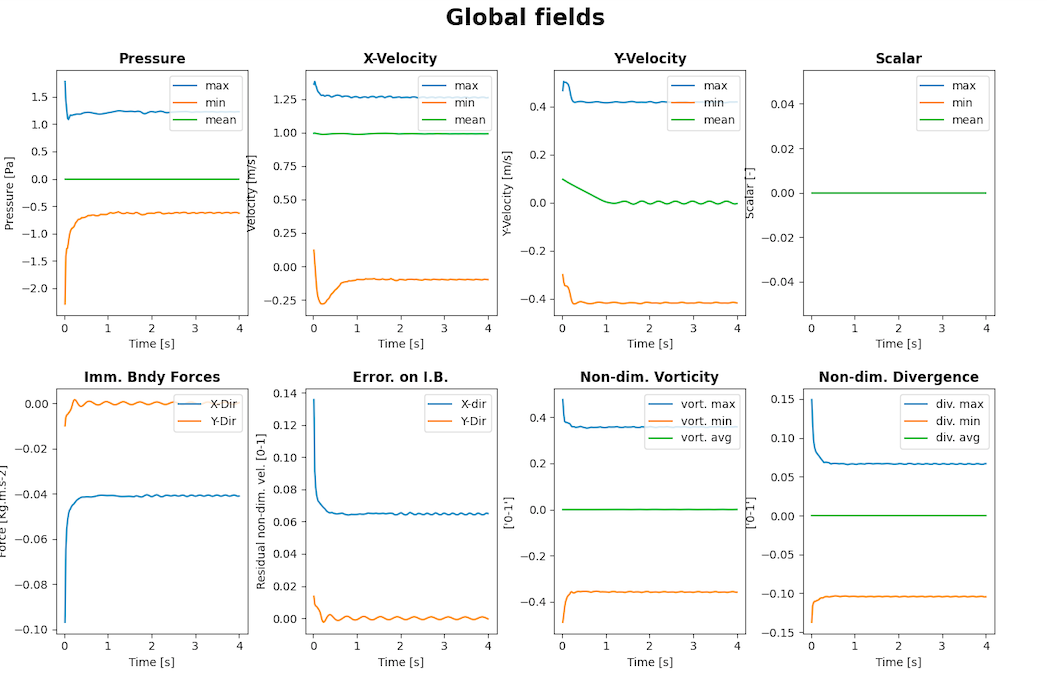karmanmonitor

The solver monitoring gives the following data in terms of time:

• pressure
• x-velocity
• y-velocity
• scalar
• Immersed boundaries forces, for further information
• Error on Immersed boundary
• Non dimensional Vorticity
• Non dimensional Divergence

### Paraview¶

For the cylinder case, .xmf files are created and can be read with paraview. Those file are created in the time loop thanks to :

```for i in range(nsave):
dom.dump_paraview(time=time)
```

Open barbaresult.xmf in paraview :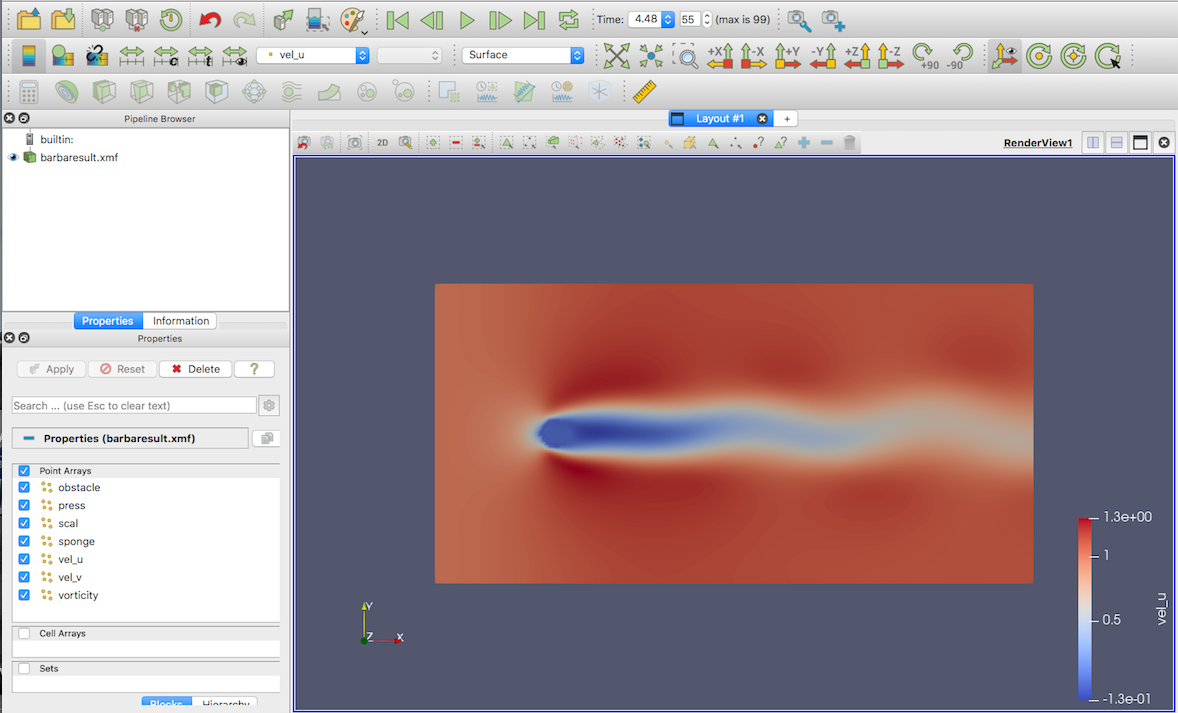paraviewcylinder